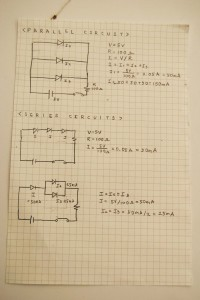# Bonus-Pcomp

for the final project in Pcomp, I used 8×8 LED matrix and Real Time Clock device.

what I used for this project is RTC, LED Matrix, two shift registers, capacitor, magnets, LED, conductive tape.

I combined with Pcomp and soft circuit so user can play with each clock and make own shape with cubes.

Each clock shows different time of location and weather.

Video

Code# Final CClab

From this final project, I have worked with pulse sensor and it was first time to make a interaction project.

It was really fun and what I learned from this project, as people are trapped in technology,

I want to provide people an opportunity for themselves to interact with hear rate which they can’t see.

Based on critique, next step is show different way with pulse sensor and add more personalized visualization.

Arduino Code

Processing Code

Presentation PDF File

Fake Motion Blur -github

this is my OF files

# Mterm Project

For the mterm project in CClab, I want to use pulse sensor to show people their heart rate.

so this time, I tried to finsih countdown code and save frame from video camera.

this is video

this is my processing code

# Data Visualization

For this project, I tried to connect with arduino and processing. Running with processing was hard because flower shape has for loop.

I used AN with range finder. first I checked sensor value and divide by 4 drawing.

Each one draws different shape.

<Video>

Range Finder Test

Arduino+Processing

Screen# Pulse Sensor

Hyungee Koo, Julie Lee, Norihito YukiWe used a pulse sensor Julie bought at Maker Fair. Pulse sensor consists of  a led and a photocell as a detector. You can see how a pulse sensor works from this site.

Video

Based on what I learned in CClab and Pcom, I made a fortune teller – arduino by myself.

While I was working on this, I almost gave up but i tried to connect again from the first part.

I am so happy that this project is exactly what I want to do at the first time.

I used 8×8 dot matrix to show emoticon.

there are 3 option (I used random function) led1, led2, and led3.

Once it start I used buttonPin which is pin7. if this button state is high, it generate random number (0 to 2)

this is my code.

vimeo video – fortune teller1

vimeo video – fortune teller2

this is the website that I convert to hexadecimal here

I found this drawing pixel generator website

int randNumber;
int val = 0;
int buttonPin = 7;
//Pin connected to ST_CP of 74HC595
int latchPin = 8;
//Pin connected to SH_CP of 74HC595
int clockPin = 12;
//Pin connected to DS of 74HC595
int dataPin = 11;
int count=0;
int led1 = 3;
int led2 = 5;
int led3 = 10;
byte test;
byte drawing1;
byte drawing2;
byte drawing3;
void setup(){
pinMode(buttonPin, INPUT);
Serial.begin(9600);
pinMode(led1, OUTPUT);
pinMode(led3, OUTPUT);
pinMode(led3, OUTPUT);
pinMode(latchPin, OUTPUT);
pinMode(clockPin, OUTPUT);
pinMode(dataPin, OUTPUT);
test=0x7F; //01111111
test=0xBF; //10111111
test=0xDF; //11011111
test=0xEF; //11101111
test=0xF7; //11110111
test=0xFB; //11111011
test=0xFD; //11111101
test=0xFE; //11111110
drawing1=0×66;
drawing1=0xF7;
drawing1=0x7F;
drawing1=0x3E;
drawing1=0x7C;
drawing1=0xFE;
drawing1=0xEF;
drawing1=0×66;
drawing2=0×66;
drawing2=0xF7;
drawing2=0x7F;
drawing2=0x3E;
drawing2=0x7C;
drawing2=0xFE;
drawing2=0xEF;
drawing2=0×66;
drawing3=0×66;
drawing3=0xF7;
drawing3=0x7F;
drawing3=0x3E;
drawing3=0x7C;
drawing3=0xFE;
drawing3=0xEF;
drawing3=0×66;
}
void loop() {
Serial.println(randNumber);
digitalWrite(led1, LOW);
digitalWrite(led2, LOW);
digitalWrite(led3, LOW);
randNumber = random(1000)%3;
if (val == HIGH) {
if (randNumber == 0){
digitalWrite(led1, HIGH);
}
if (randNumber == 1){
digitalWrite(led2, HIGH);
}
if(randNumber == 2){
digitalWrite(led3, HIGH);
}
}
if(val == LOW){
digitalWrite(led1, LOW);
digitalWrite(led1, LOW);
digitalWrite(led1, LOW);
}
Serial.println(randNumber);
}

# Assignment5

I uploaded 3 videos in vimeo

Parallel Circuit

Series Circuit

Parallel+Series circuit

Schematics + CalculationsIf I calculate with LED resistor,

every LED has resistor and my LED resistor is 3V so

1. Current of first parallel circuit will be

5v-3v=2v

I=V/R

I=2v/100ohm

20mA+20mA+20mA=60mA

2. current of second series circuit will be 20mA.

3. current of third parallel and series circuit will be

I=20mA

I2=10mA

I3=10mA

I am taking Physical Computing so it was not really new and it is fun. From Pcom class everyone is good at arduino and the progress of class is fast

so I had hardtime to catch up. I think this cclab with arduino will really helpful to understand circuit.

Also I haven’t calculated ohm’s law before so it makes me to understand more about resistor voltage and current.